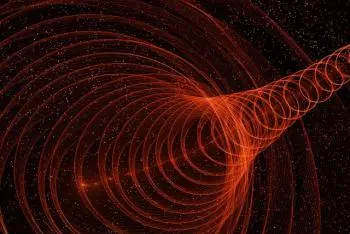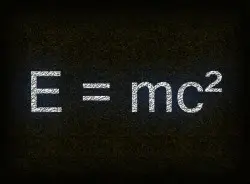# Albert Einstein's Theory of Relativity - Special and GeneralThe Theory of Relativity, formulated by German physicist Albert Einstein in the early 20th century, revolutionized our understanding of the universe and forever changed the way we perceive space, time and gravity.

This theory, divided into two parts, Special Relativity and General Relativity, has been one of the fundamental pillars of modern physics and has given rise to countless scientific and technological advances.

## Theory of Special Relativity

The first part of the Theory of Relativity, Special Relativity, was published by Einstein in 1905. This theory addresses problems related to uniform motion and establishes two fundamental postulates:

• Principle of relativity : The laws of physics are the same for all observers moving at constant speeds relative to each other. In other words, physical laws are universal and do not depend on the speed of an observer.
• Constant speed of light : The speed of light in a vacuum is constant and is the same for all observers, regardless of their relative motion.

These two postulates led to the formulation of revolutionary concepts, such as time dilation and length contraction.

In short, Special Relativity predicts that time and space are relative and depend on the speed of the observer. When an object moves at speeds close to the speed of light, time slows down for that object, and its length in the direction of motion contracts.

## General Relativity TheoryThe second part of the Theory of Relativity, General Relativity, was published by A. Einstein in 1915. This theory is an extension of Special Relativity and addresses the influence of gravity on the geometry of space-time.

Instead of viewing gravity as a force, as Newton's theory of gravitation did, General Relativity describes gravity as the curvature of space-time caused by the presence of mass and energy.

The pillars of General Relativity are:

• Principle of Equivalence : The acceleration due to gravity is indistinguishable from the acceleration due to an accelerated reference frame. This means that an observer in a gravitational field cannot distinguish whether it is at rest in a gravitational field or accelerating in free space.
• Einstein Field Equations : These equations relate the curvature of space-time to the distribution of mass and energy in the universe. They are the equivalent of Newton's equations for gravity, but in the context of General Relativity.

General Relativity has been confirmed by astronomical observations and experiments on Earth, such as the famous experiment confirming the bending of starlight during a solar eclipse in 1919.

This theory has had a profound impact on astronomy and cosmology, allowing the prediction and understanding of phenomena such as black holes, the expansion of the universe and gravitational waves.

## Einstein Energy EquationEinstein's famous energy equation, E=mc², is one of the most iconic equations in physics and is a direct consequence of the Theory of Special Relativity. This equation relates three fundamental concepts: energy (E), mass (m) and the speed of light in a vacuum (c).

• E: Represents energy. Energy and mass are related in a surprising new way. Energy is not something completely separate from mass, but can be converted into mass and vice versa.

• m: Represents the mass. Mass is a measure of how much matter an object contains. Traditionally, mass was thought to be constant and unchanging. However, Einstein proposed that the mass of an object can change when it moves at speeds close to the speed of light.

• c: Represents the speed of light in a vacuum, which is a universal constant. Its value is approximately 299,792,458 meters per second. The speed of light in a vacuum is the maximum speed at which any information or energy can travel in the universe, according to the Theory of Relativity.

The equation E=mc² states that energy (E) is equal to mass (m) multiplied by the square of the speed of light (c²). This means that energy and mass are proportionally related: as the speed of light is a very large constant, even a small amount of mass can be converted into a large amount of energy and vice versa.

This equation was fundamental in the understanding of nuclear processes and the energy released in nuclear reactions. In nuclear fission reactions that occur in a nuclear reactor in which an atom is divided, there is a loss of mass of the resulting products that is converted into energy.

## Impact on Science and Society

The Theory of Relativity has transformed the way we understand the cosmos and has driven a large number of scientific and technological advances. Some of the most notable impacts include:

### Astronomy and CosmologyGeneral Relativity has opened new doors in the exploration of cosmic objects, such as black holes and singularities, and has provided a solid foundation for modern cosmology, including the Big Bang theory.

### Accurate GPS

Without the correction for time dilation predicted by Special Relativity, global positioning systems (GPS) would not function with the precision we enjoy today.

### Nuclear EnergyEinstein's famous equation, E=mc², is a direct consequence of Special Relativity and has been fundamental to the understanding of the energy released in nuclear reactions, such as those that occur in a nuclear reactor, the sun, and atomic bombs.

### Technological Development

Research in theoretical physics has driven advances in technologies such as nuclear energy, space exploration, and gravitational wave detection.

Author:

Published: September 6, 2023
Last review: September 6, 2023